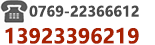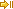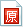﻿ Winform开发框架 - 使用Linq判断日期时间范围查询条件的方法|C/S框架网C/S框架网 | 网站导航 | C/S框架文档 - 综合区 | Winform开发框架 - 使用Linq判断日期时间范围查询条件的方法
 Winform开发框架 - 使用Linq判断日期时间范围查询条件的方法 作者:C/S框架网|www.csframewo  发布日期:2023/03/06 16:31:31Winform开发框架 - 使用Linq判断日期时间范围查询条件的方法C# Code: var q = _Database.GetQueryable();if (!input.DocType.IsEmpty())q = q.Where(w => w.DocType.CompareTo(input.DocType) == 0);if (!input.DocNo.IsEmpty())q = q.Where(w => w.DocNo.CompareTo(input.DocNo) == 0);if (!input.ImageType.IsEmpty())q = q.Where(w => w.ImageType.CompareTo(input.ImageType) == 0);if (!input.Title.IsEmpty())q = q.Where(w => w.Title.Contains(input.Title));//开始时间 if (input.DocDateFrom > Globals.MinSqlDate)//1900-01-01 {var time1 = input.DocDateFrom.ToMinTime();q = q.Where(w => w.CreationDate >= time1);}//结束时间 if (input.DocDateTo > Globals.MinSqlDate)//1900-01-01 {var time2 = input.DocDateTo.ToMaxTime();q = q.Where(w => w.CreationDate <= time2);}return q.ToList();//来源:C/S框架网 | www.csframework.com | QQ:23404761扫一扫加作者微信